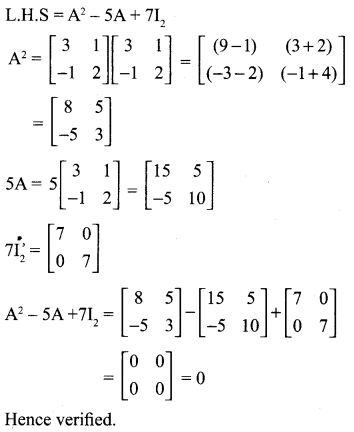## Tamilnadu Samacheer Kalvi 10th Maths Solutions Chapter 3 Algebra Ex 3.18

10th Maths Exercise 3.18 Answers Question 1.
If A is of order p × q and B is of order q × r what is the order of AB and BA?
Solution:
If A is of order p × q [∵ p × q q × r = p × r]
the order of AB = p × r [∵ q × r p × q = r ≠ p]
Product of BA cannot be defined/found as the number of columns in B ≠. The number of rows in A.

10th Maths Exercise 3.18 Question 2.
If A is of order p × q and B is of order q × r what is the order of AB and BA?
Order of A = a × (a + 3)
Order of B = b × (17 – b)
Given: Product of AB exist
a + 3 = b
a – b = – 3 ….(1)
Product of BA exist
17 – b = a
– a – b = -17
a + b = 17 ………(2)
(1) + (2) ⇒ 2a = 14
a = $$\frac { 14 }{ 2 }$$ = 7
Substitute the value of a = 7 in (1)
7 – b = -3 ⇒ -b = -3 -7
-b = -10 ⇒ b = 10
The value of b = 7 and b = 10

Ex 3.18 Class 10 Question 3.
Find the order of the product matrix AB if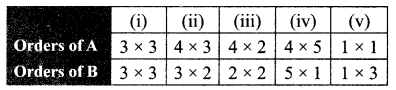Solution: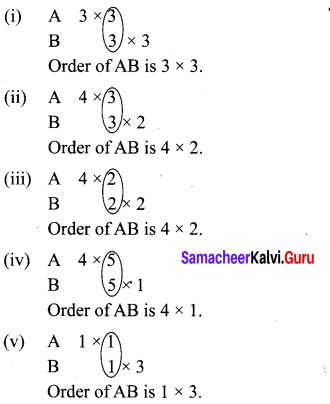10th Maths Exercise 3.18 Samacheer Kalvi Question 4.
If A = $$\left[\begin{array}{ll}{2} & {5} \\ {4} & {3}\end{array}\right]$$, B = $$\left[\begin{array}{cc}{1} & {-3} \\ {2} & {5}\end{array}\right]$$ find AB, BA and check if AB = BA?
Solution: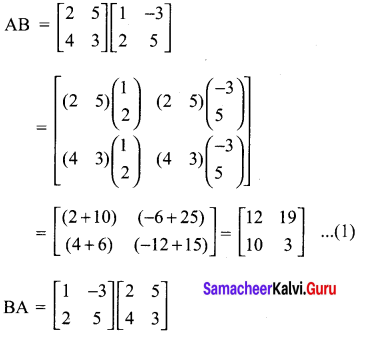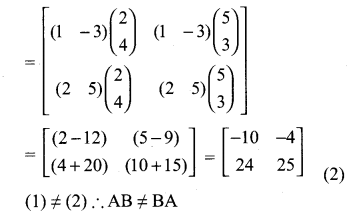Exercise 3.18 Class 10 Question 5.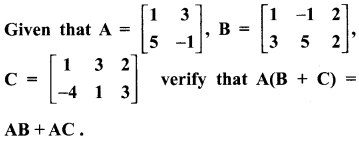Solution: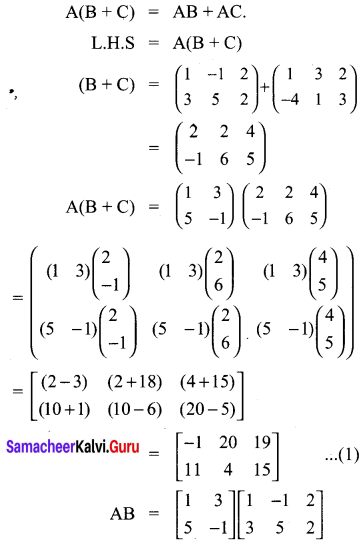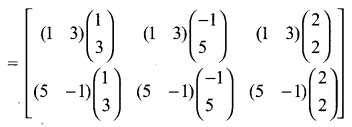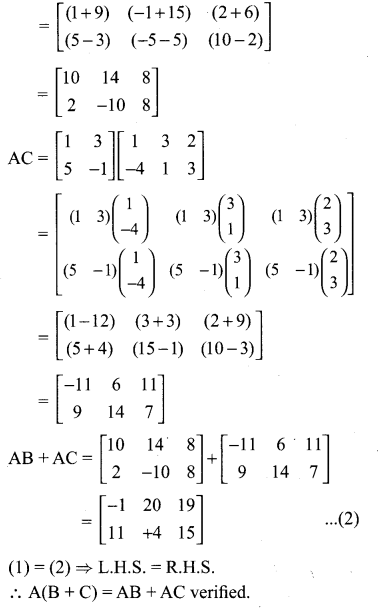Ex 3.18 Class 10 Samacheer Question 6.
Show that the matrices A = $$\left[\begin{array}{ll}{1} & {2} \\ {3} & {1}\end{array}\right]$$, B = $$\left[\begin{array}{cc}{1} & {-2} \\ {-3} & {1}\end{array}\right]$$ satisfy commutative property AB = BA
Solution: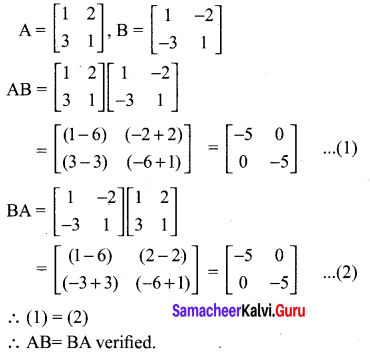10th Maths Exercise 3.18 In Tamil Question 7.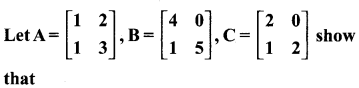(i) A(BC) = (AB)C
(ii) (A – B)C = (AC – BC)
(iii) (A- B)T = AT – BT
Solution:
(i) A(BC) = (AB)C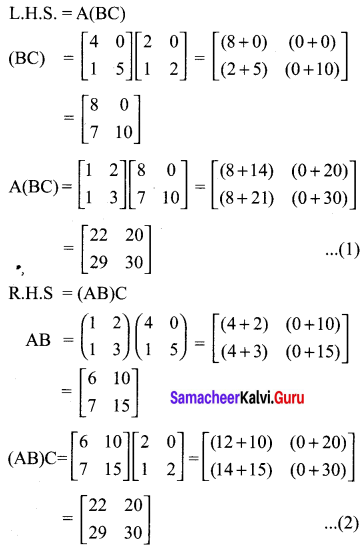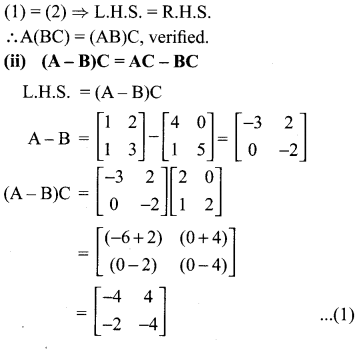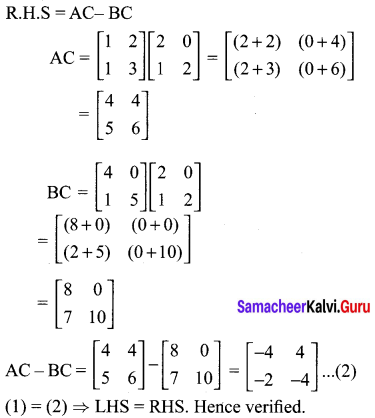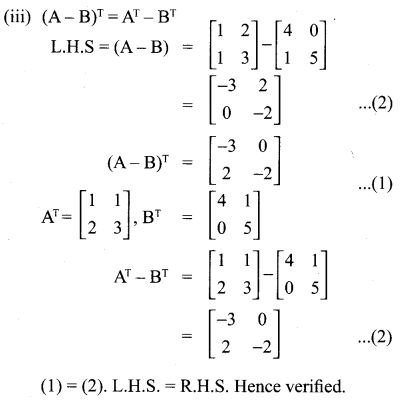10th New Syllabus Maths Exercise 3.18 Question 8.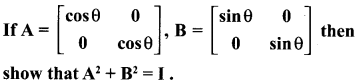Solution: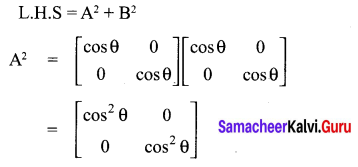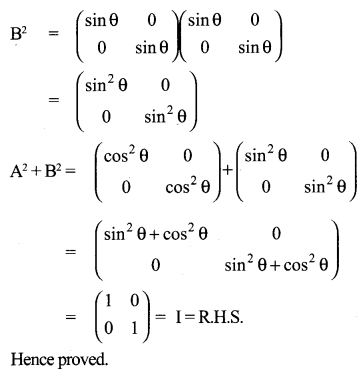10th Maths Exercise 3.18 3rd Sum Question 9.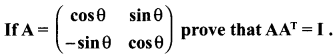Solution: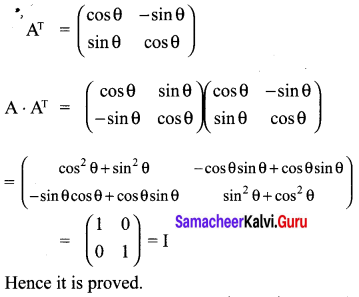10th Maths 3.18 Question 10.
Verify that A2 = I when A = $$\left(\begin{array}{cc}{5} & {-4} \\ {6} & {-5}\end{array}\right)$$
Solution: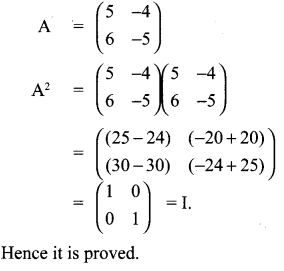Samacheer Kalvi 10th Maths Graph Question 11.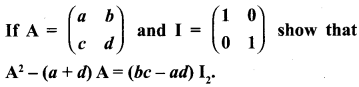Solution: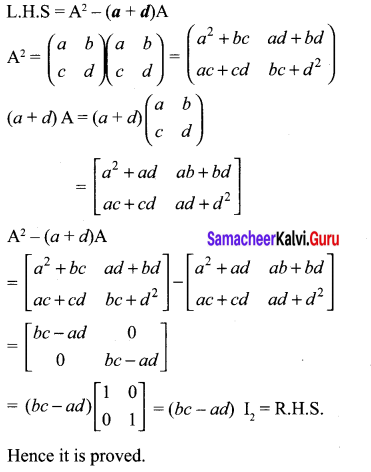Samacheer Kalvi Guru 10th Maths Question 12.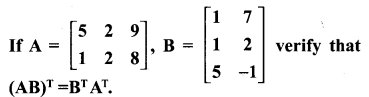Solution: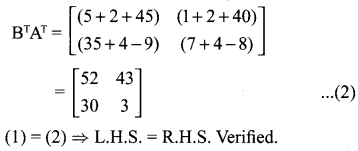Samacheerkalvi.Guru 10th Maths Question 13.
If A =  show that A2 – 5A + 7I2 = 0.
Solution: